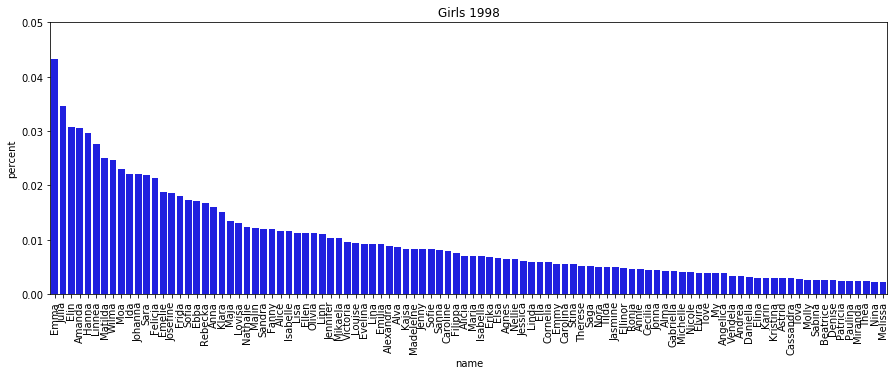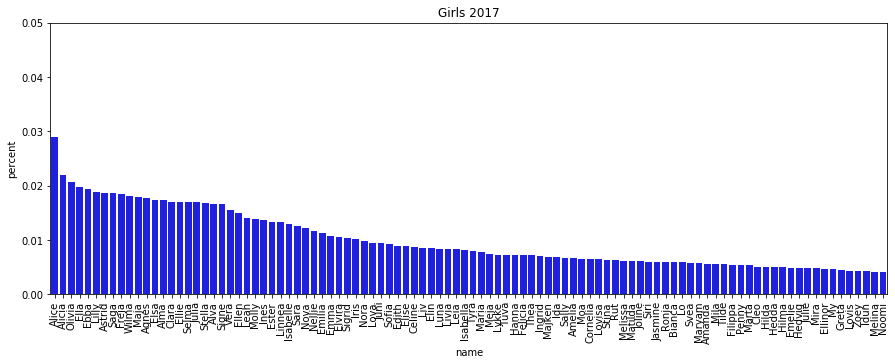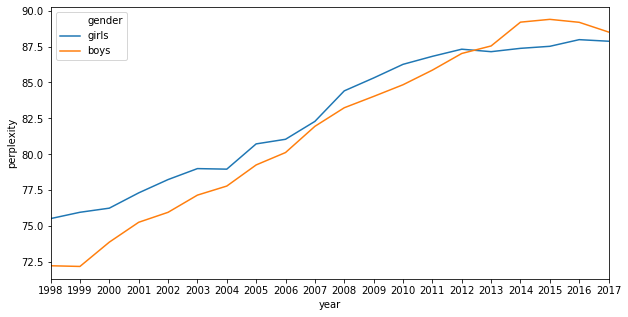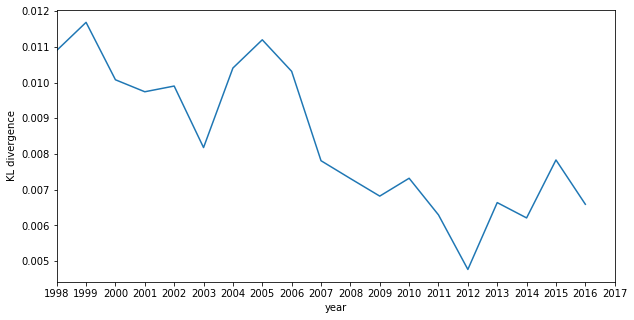# Are parents picking less common names?

I think we all have the gut feeling that parents nowadays try to pick more unique names, or at least not names that are too common. Instead of relying on a gut feeling or anectdata, let's check!

Being Swedish, of course I'm mostly interested in Swedish name statistics, and luckily SCB (the central statistics bureau) provides an Excel spread sheet with the frequency statistics of the top 100 names for boys and girls from 1998 to 2017. Let's download it:

! wget -O stats.xlsx https://www.scb.se/hitta-statistik/statistik-efter-amne/befolkning/amnesovergripande-statistik/namnstatistik/pong/tabell-och-diagram/nyfodda--efter-namngivningsar-och-tilltalsnamn-topp-100/Namn-1998-/


Now we can read the spreadsheet with Python and pandas/numpy (I bet it would be easier to just do this in Excel, but I'm not an Excel-ninja...) and collect the statistics.

Let's load the Excel file and look at the data:

import pandas as pd
import numpy as np

START_YEAR, END_YEAR = 1998, 2017
GENDERS = ['girls', 'boys']

data = pd.DataFrame(columns=['year', 'name', 'gender', 'count', 'percent'])

f = open('stats.xlsx', 'rb')
for year in range(START_YEAR, END_YEAR+1):
for gender_idx, gender in enumerate(GENDERS):
sheet_idx = 2*(year - START_YEAR) + gender_idx + 1
count_col = 'Antal' if 'Antal' in df.columns else 'Antal bärare'
counts = df[count_col].values[1:]
name_col_idx = list(df.columns).index(count_col) - 1
names = df.iloc[1:, name_col_idx].str.strip() # NOTE: need to strip spaces
# When there are ties in count, there will be more than 100 rows. Keep the top 100
counts, names = counts[:100], names[:100]
years = [year]*len(counts)
genders = [gender]*len(counts)
data = data.append(
pd.DataFrame(data={'year': years, 'gender': genders, 'name': names, 'count': counts,
'percent': counts.astype(float) / counts.sum()}),
sort=True)

print(data)

    count gender    name   percent  year
1    1468  girls    Emma  0.043336  1998
2    1171  girls   Julia  0.034568  1998
3    1043  girls    Elin  0.030790  1998
4    1037  girls  Amanda  0.030613  1998
5    1006  girls   Hanna  0.029697  1998
..    ...    ...     ...       ...   ...
96    145   boys    Thor  0.004173  2017
97    142   boys  Milian  0.004087  2017
98    141   boys    Levi  0.004058  2017
99    141   boys    Vide  0.004058  2017
100   139   boys     Neo  0.004000  2017

[4000 rows x 5 columns]


Let's plot what the top 100 names looked like in 1998 and 2017:

import seaborn as sns
import matplotlib.pyplot as plt

for year in [1998, 2017]:
fig, ax = plt.subplots(figsize=(15, 5))
girls_year = data[(data['year'] == year) & (data['gender'] == 'girls')]
ax = sns.barplot(x='name', y='percent', data=girls_year,
color='blue')
ax.set_xticklabels(ax.get_xticklabels(), rotation=90);
ax.set(ylim=(0, 0.05));
ax.set_title(f'Girls {year}');These distributions look quite different, but the question is what's a good measure for the unevenness of the name distribution so that we can compare different years. I'm sure there are many options for this, but I'll pick perplexity which is the exponentiated entropy. The entropy of the distribution measures the disorder, i.e. we get the highest entropy if all names have an equal probability, and low entropy if everyone named their child the same way. Perplexity can be seen as the average number of "choices" one has in the random variable, e.g. a 6-sided fair die has a perplexity of 6, while a cheating die that always ends up on one number has a perplexity of 1. This is a bit easier to interpret than entropy, which is the average number of bits needed to encode an event from that distribution.

Let's calculate the perplexity over the name distribution for each year and gender and plot it over time:

perplexity_data = pd.DataFrame(columns=['year', 'perplexity', 'gender'])
for year in range(START_YEAR, END_YEAR+1):
for gender in GENDERS:
counts = data.loc[(data['year'] == year) & (data['gender'] == gender), 'count'].astype(float)
probs = counts / counts.sum()
entropy = -(probs * np.log(probs)).sum()
perplexity = np.exp(entropy)
perplexity_data = perplexity_data.append(
{'year': year, 'perplexity': perplexity, 'gender': gender}, ignore_index=True)

fig, ax = plt.subplots(figsize=(10, 5))
ax.set_xlim(START_YEAR, END_YEAR)
ax.set_xticks(range(START_YEAR, END_YEAR+1))
ax = sns.lineplot(x='year', y='perplexity', hue='gender', data=perplexity_data)It seems like within the top 100 names, the answer is an unequivocal yes! Parents are spreading out their name choices more and more, approaching 90/100 which is quite even.

Another interesting thing to check is whether names comes into, and goes out of fashion quicker over the years. My intuition tells me that due to naming lists being published online etc, this might be the case. One way we could check is to measure some kind of difference between the name distributions between adjacent years. One measure we could use is the KL-divergence, which tells us in a sense how different one distribution is from another expected distribution:

kl_divs = []
for year1 in range(START_YEAR, END_YEAR):
year2 = year1 + 1
data_year1 = data.loc[data['year'] == year1]
data_year2 = data.loc[data['year'] == year2]
names = list(set(data_year1['name']) & set(data_year2['name']))
name_stats_year1 = data_year1.loc[data_year1['name'].isin(names), ['name', 'count']]
name_stats_year2 = data_year2.loc[data_year2['name'].isin(names), ['name', 'count']]
name_stats_year1 = name_stats_year1.sort_values('name')
name_stats_year2 = name_stats_year2.sort_values('name')
q = name_stats_year1['count'].to_numpy().astype(float)
q = q / q.sum()
p = name_stats_year2['count'].to_numpy().astype(float)
p = p / p.sum()
kl_div = -(p*np.log(q/p)).sum()
kl_divs.append(kl_div)

fig, ax = plt.subplots(figsize=(10, 5))
ax.set_xlim(START_YEAR, END_YEAR)
ax.set_xticks(range(START_YEAR, END_YEAR+1))
kl_div_data = pd.DataFrame(data={
'year': list(range(START_YEAR, END_YEAR)), 'KL divergence': kl_divs})
ax = sns.lineplot(x='year', y='KL divergence', data=kl_div_data)Surprisingly, for me at least, it seems intra-year KL divergence has gone down, but come to think of it, it makes sense. In 1998 when the distribution was more uneven, naming trends (e.g. a popular name waning) would have a large impact on the naming distribution, while a more even distribution suggests smaller changes between years.